## 1　Helmholtz coil

This is a calculation of the magnetic field created in space only by the coil using a Helmholtz coil.## 2　U-shaped magnet

Calculate the magnetic field and magnetic field contours created in space by the magnet alone using a U-shaped magnet.## 3　Halbach magnet

Calculate the magnetic field and magnetic field contours created in space by the magnet alone using a Halbach magnet.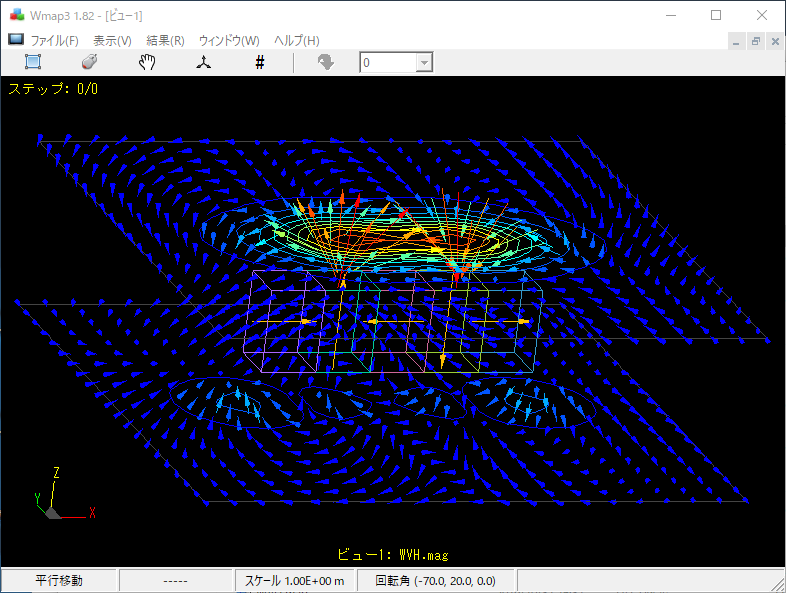## 4　Force acting on magnets

Calculate the force acting on magnets using Maxwell stresses.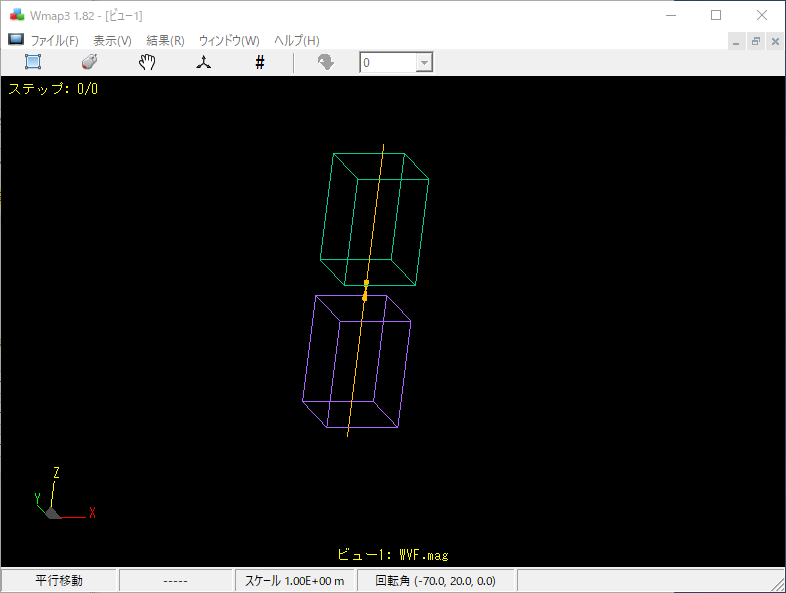## 5　Coil and magnetic material

Perform a general ELF/MAGIC calculation where the coil is wound around a magnetic material.## 6　Eddy current insulation and conduction

Vary the current in the coil and calculate the eddy currents flowing in the conductor plates.## 7　Eddy current hole model

Vary the current in the coil and calculate the eddy currents flowing in the conductor plates.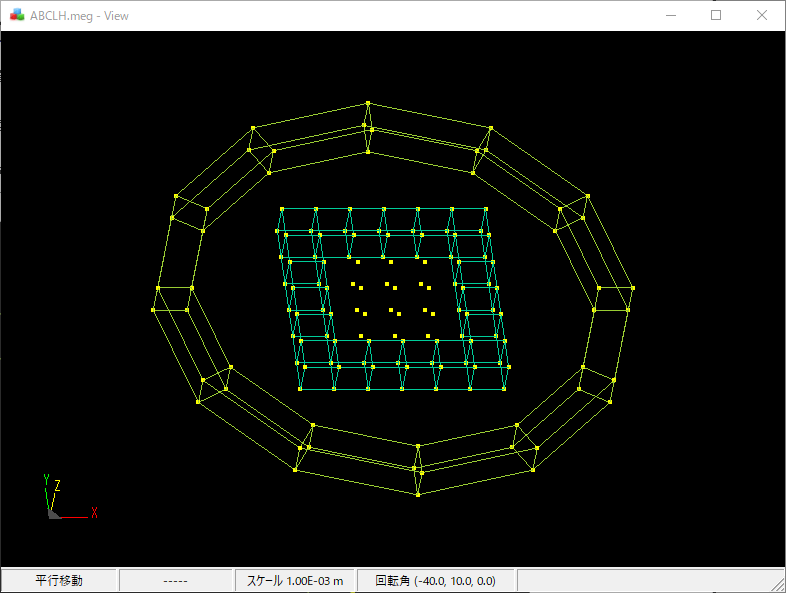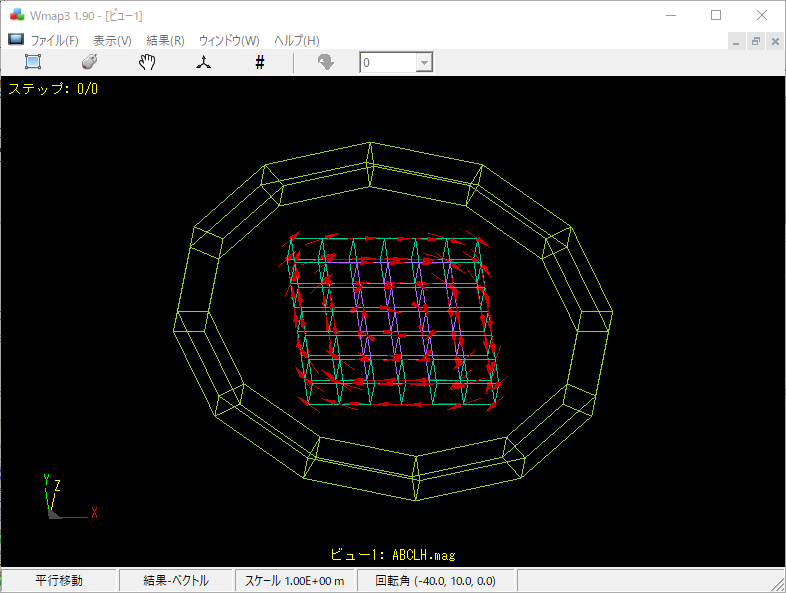## 8　Induced current

Calculate the case of induced current in coils.Here is an example of calculating the interlinkage flux of coils.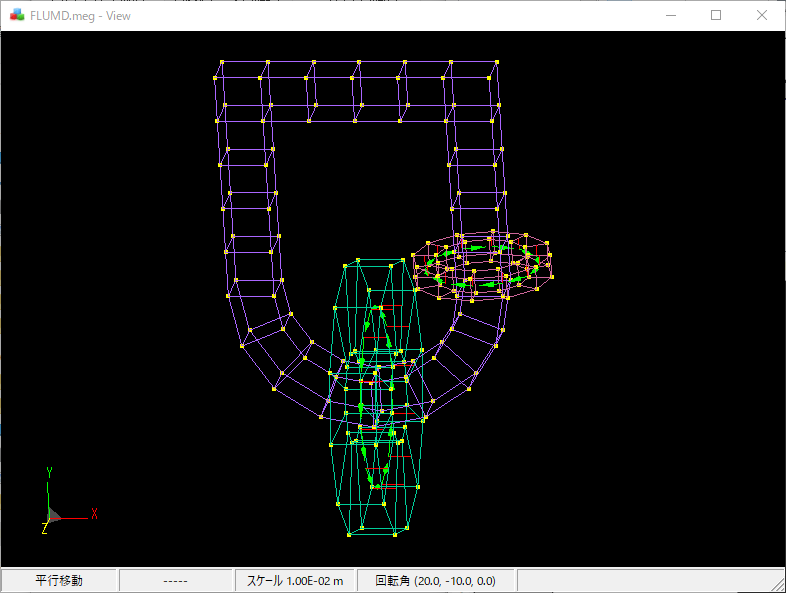## 10　Coils and magnetic materials 2

This is a continuation of the example calculation where a coil is wound around a magnetic material. Calculate the induced current.## 11　Iron balls and magnets

Magnetic surfaces of arbitrary shape can be represented by triangular or rectangular elements for calculation.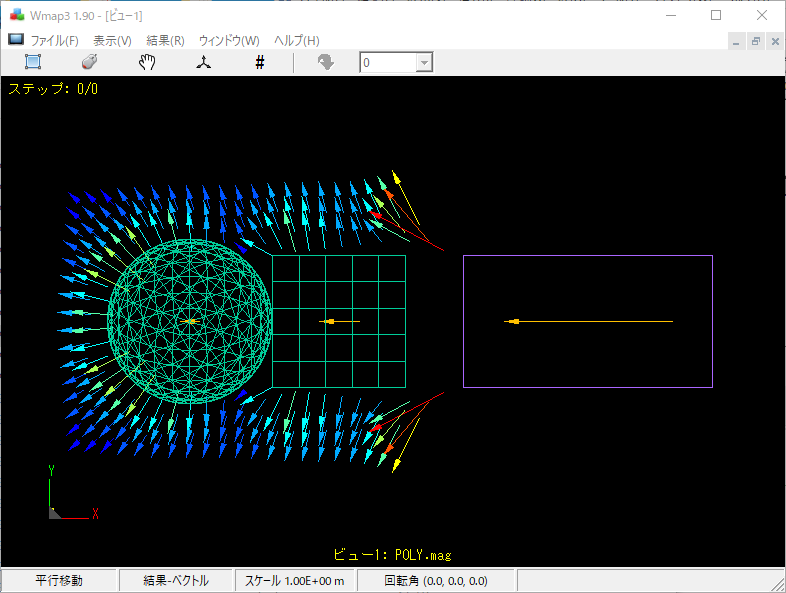a:2508 t:1 y:0